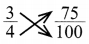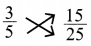# DAV Class 4 Maths Chapter 9 Worksheet 4 Solutions

The DAV Class 4 Maths Solutions and DAV Class 4 Maths Chapter 9 Worksheet 4 Solutions of Fractions offer comprehensive answers to textbook questions.

## DAV Class 4 Maths Ch 9 WS 4 Solutions

Question 1.
Find if the following pairs of fractions are equivalent.
(a) $$\frac{1}{3}$$ and $$\frac{3}{9}$$
$$\frac{1}{3}$$ and $$\frac{3}{9}$$1 × 9 = 9
3 × 3 = 9
so, $$\frac{1}{3}=\frac{3}{9}$$

(b) $$\frac{2}{5}$$ and $$\frac{8}{25}$$2 × 25 = 50
5 × 8 = 40
so, $$\frac{2}{5} \neq \frac{8}{25}$$

(c) $$\frac{10}{15}$$ and $$\frac{2}{3}$$10 × 3 = 30
15 × 2 = 30
so, $$\frac{10}{15}>\frac{2}{3}$$

(d) $$\frac{3}{7}$$ and $$\frac{24}{56}$$3 × 56 = 168
7 × 24 = 168
so, $$\frac{3}{7}=\frac{24}{56}$$

(e) $$\frac{1}{10}$$ and $$\frac{1}{40}$$10 × 1 = 10
1 × 40 = 40
so, $$\frac{1}{10} \neq \frac{1}{40}$$

(f) $$\frac{3}{4}$$ and $$\frac{75}{100}$$3 × 100 = 300
4 × 75 = 300
so, $$\frac{3}{4}=\frac{75}{100}$$

(g) $$\frac{2}{9}$$ and $$\frac{18}{81}$$2 × 81 = 162
9 × 18 = 162
so, $$\frac{2}{9}=\frac{18}{81}$$

(h) $$\frac{15}{16}$$ and $$\frac{30}{48}$$15 × 48 = 720
16 × 30 = 480
so, $$\frac{15}{16} \neq \frac{30}{48}$$

(i) $$\frac{7}{11}$$ and $$\frac{49}{77}$$7 × 77 = 539
11 × 49 = 539
so, $$\frac{7}{11}=\frac{49}{77}$$Question 2.
Write ‘True’ or ‘False’ for the following:
(a) $$\frac{7}{8}=\frac{35}{40}$$
True
7 × 40 = 280
8 × 35 = 280

(b) $$\frac{1}{5}=\frac{8}{50}$$
False
1 × 50 = 50
5 × 8 = 40

(c) $$\frac{11}{13}=\frac{22}{26}$$
True
11 × 26 = 286
13 × 22 = 286

(d) $$\frac{25}{30}=\frac{5}{6}$$
True
25 × 6 = 150
30 × 5 = 150

(e) $$\frac{100}{700}=\frac{10}{70}$$
True
100 × 70 = 7000
700 × 10 = 7000

(f) $$\frac{12}{9}=\frac{9}{12}$$
False
12 × 12 = 144
9 × 9 = 81

### DAV Class 4 Maths Chapter 9 Worksheet 4 Notes

If the cross products of numerator of one and denominator of the other fraction are same, the two fractions are equivalent.
Example:i.e 3 × 25 = 75
5 × 15 = 75
so, $$\frac{3}{5}=\frac{15}{25}$$